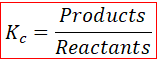# Problem: When the following reaction comes to equilibrium, will the concentrations of the reactants or products be greater?A(g) + B(g) ⇌ 2 C(g); Kc = 1.4 x 10–5

###### FREE Expert Solution

Chemical equilibrium is a state in which the rate of forward reaction equals the backward reactionThe equilibrium constant K is a number that is equal to the ratio of products to reactants at a given temperature.

The rate equation or the equilibrium expression can be written as,A(g) + B(g) ⇌ 2 C(g)    Kc = 1.4 x 10–5

80% (143 ratings)###### Problem Details

When the following reaction comes to equilibrium, will the concentrations of the reactants or products be greater?
A(g) + B(g) ⇌ 2 C(g); Kc = 1.4 x 10–5